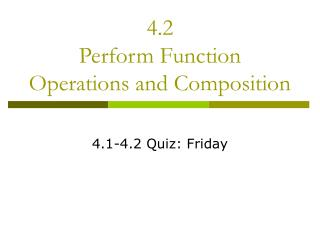DownloadDownload Presentation4.2 Perform Function Operations and Composition

# 4.2 Perform Function Operations and Composition

Télécharger la présentation## 4.2 Perform Function Operations and Composition

- - - - - - - - - - - - - - - - - - - - - - - - - - - E N D - - - - - - - - - - - - - - - - - - - - - - - - - - -
##### Presentation Transcript

1. 4.2Perform Function Operations and Composition 4.1-4.2 Quiz: Friday

2. Vocabulary • Power function • A function of the form y = axb where a is a real number and b is a rational number • Composition • The composition of a function g with a function f is h(x) = g(f(x)). • Composition is a weird way of saying SUBSTITUE

3. Example 1: • Let f(x) = 3x2 and g(x) = -5x2. Find the following. • f(x) + g(x) • f(x) – g(x)

4. You Try: • Let f(x) = 4x3 and g(x) = -2x3. Find the following. • g(x) + f(x) • f(x) – g(x)

5. Example 2: • Let f(x) = 7x and g(x) = x6. Find the following. • f(x) • g(x) • f(x) ÷ g(x)

6. You Try: • Let f(x) = 4x3 and g(x) = -2x3. Find the following. • f(x) • g(x) • g(x) ÷ f(x)

7. Example 3: • Let f(x) = 6x-1 and g(x) = 3x + 5. Find the following. • f(g(x)) • g(f(x)) • f(f(x))

8. You Try: • Let f(x) = 5x – 4 and g(x) = 3x-1. Find the following. • f(g(x)) • g(f(x)) • f(f(x))